# Lesson 36 Quadratic Trigonometric Equations Pre Calculus 212022

• Slides: 21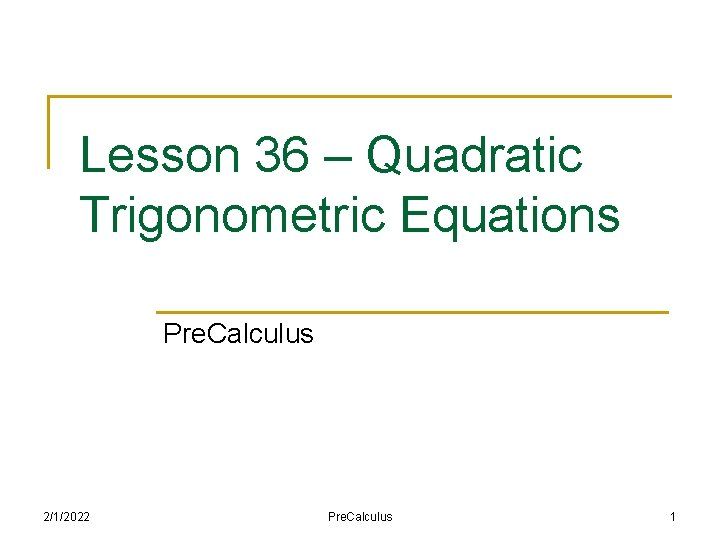Lesson 36 – Quadratic Trigonometric Equations Pre. Calculus 2/1/2022 Pre. Calculus 1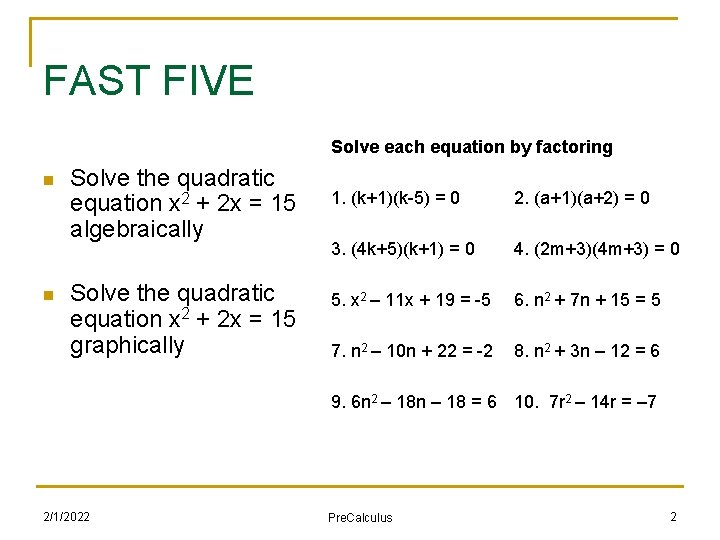FAST FIVE Solve each equation by factoring n n Solve the quadratic equation x 2 + 2 x = 15 algebraically Solve the quadratic equation x 2 + 2 x = 15 graphically 1. (k+1)(k-5) = 0 2. (a+1)(a+2) = 0 3. (4 k+5)(k+1) = 0 4. (2 m+3)(4 m+3) = 0 5. x 2 – 11 x + 19 = -5 6. n 2 + 7 n + 15 = 5 7. n 2 – 10 n + 22 = -2 8. n 2 + 3 n – 12 = 6 9. 6 n 2 – 18 n – 18 = 6 10. 7 r 2 – 14 r = – 7 2/1/2022 Pre. Calculus 2(A) Prerequisite Skill: Factoring with Trig n Factor the following trig expressions: 2/1/2022 Pre. Calculus 3(B) Solving Quadratic Trigonometric Equations n We will outline a process by which we come up with the solution to a trigonometric equation ANALYTICALLY using ALGEBRA n We will outline a process by which we come up with the solution to a trigonometric equation GRAPHICALLY using ALGEBRA n it is important you understand WHY we carry out these steps, rather than simply memorizing them and simply repeating them on a test of quiz 2/1/2022 Pre. Calculus 4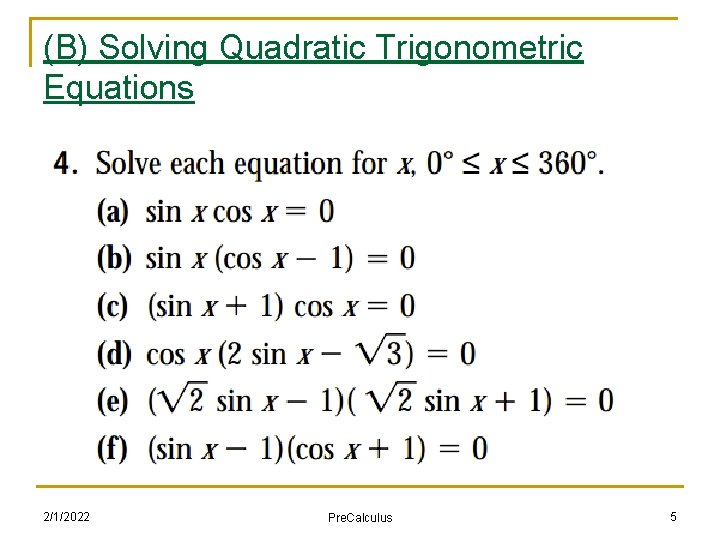(B) Solving Quadratic Trigonometric Equations 2/1/2022 Pre. Calculus 5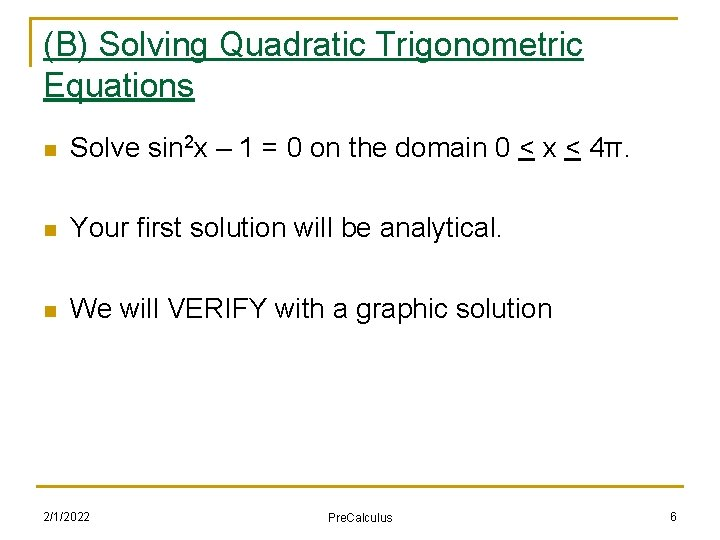(B) Solving Quadratic Trigonometric Equations n Solve sin 2 x – 1 = 0 on the domain 0 < x < 4π. n Your first solution will be analytical. n We will VERIFY with a graphic solution 2/1/2022 Pre. Calculus 6(B) Solving Quadratic Trigonometric Equations n Solve the equation 2 cos 2 x – 1 = -cosx on the domain 0 < x < 4π. n Your first solution will be analytical. n We will VERIFY with a graphic solution 2/1/2022 Pre. Calculus 7(B) Solving Quadratic Trigonometric Equations n Solve the equation 8 sin 2 x + 13 sinx = 4 – 4 sin 2 x on the domain - π < x < 3π. n Your first solution will be analytical. n We will VERIFY with a graphic solution 2/1/2022 Pre. Calculus 8(B) Solving Quadratic Trigonometric Equations n Solve 2 cos 2(θ) = 1 if 0° < θ < 360 ° 2/1/2022 Pre. Calculus 9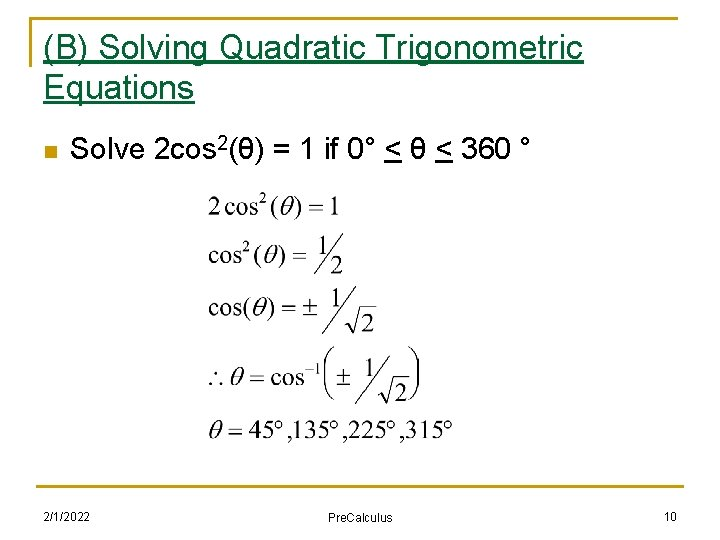(B) Solving Quadratic Trigonometric Equations n Solve 2 cos 2(θ) = 1 if 0° < θ < 360 ° 2/1/2022 Pre. Calculus 10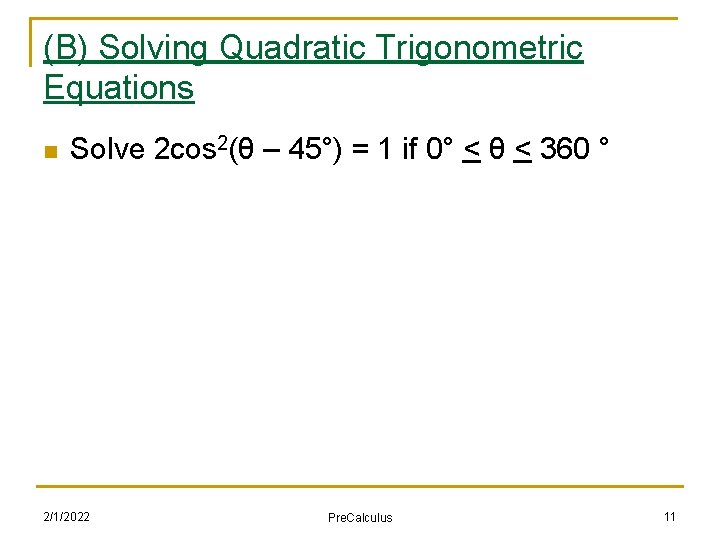(B) Solving Quadratic Trigonometric Equations n Solve 2 cos 2(θ – 45°) = 1 if 0° < θ < 360 ° 2/1/2022 Pre. Calculus 11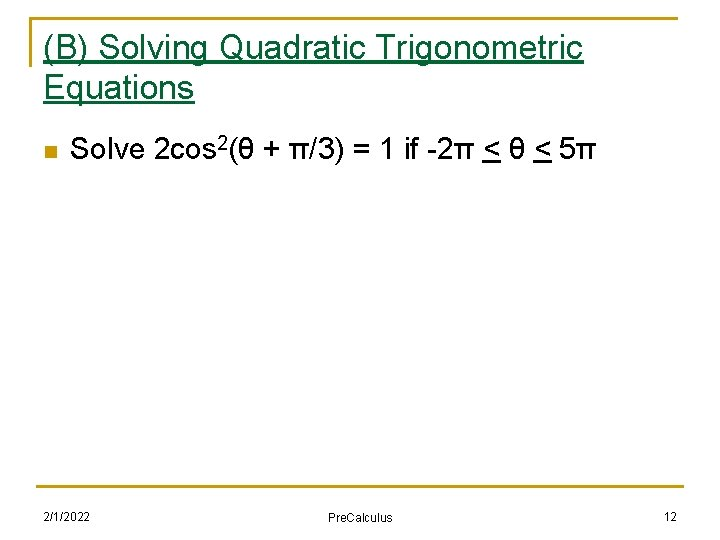(B) Solving Quadratic Trigonometric Equations n Solve 2 cos 2(θ + π/3) = 1 if -2π < θ < 5π 2/1/2022 Pre. Calculus 12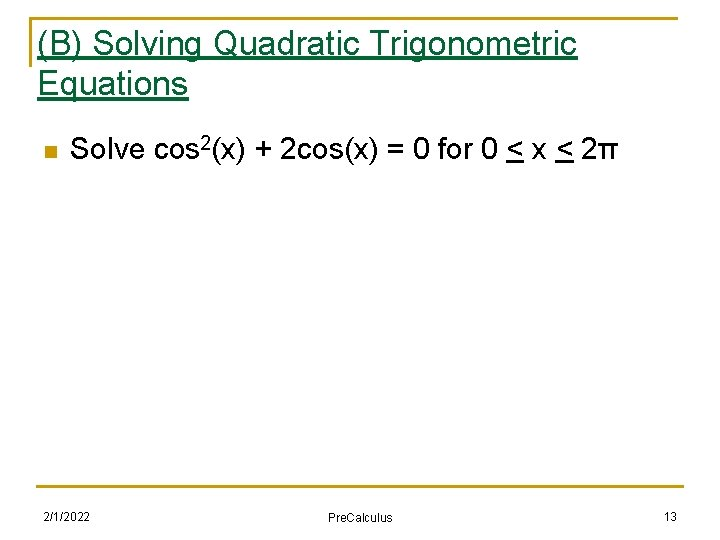(B) Solving Quadratic Trigonometric Equations n Solve cos 2(x) + 2 cos(x) = 0 for 0 < x < 2π 2/1/2022 Pre. Calculus 13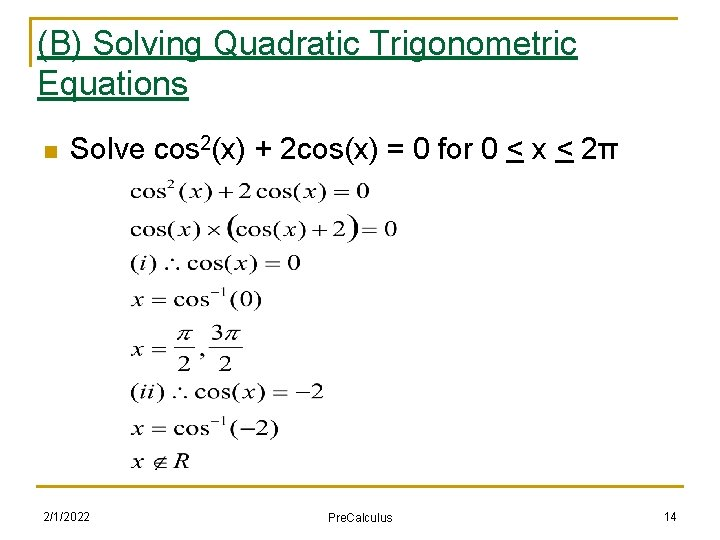(B) Solving Quadratic Trigonometric Equations n Solve cos 2(x) + 2 cos(x) = 0 for 0 < x < 2π 2/1/2022 Pre. Calculus 14(B) Solving Quadratic Trigonometric Equations n Solve cos 2(2 x) + 2 cos(2 x) = 0 for 0 < x < 2π 2/1/2022 Pre. Calculus 15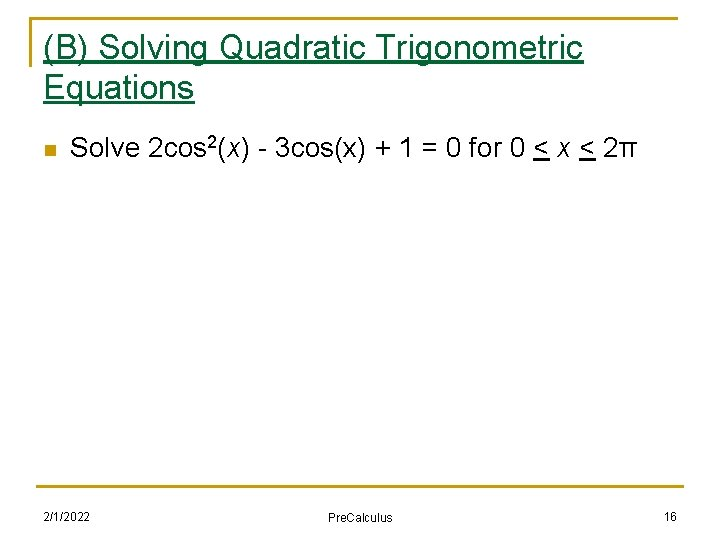(B) Solving Quadratic Trigonometric Equations n Solve 2 cos 2(x) - 3 cos(x) + 1 = 0 for 0 < x < 2π 2/1/2022 Pre. Calculus 16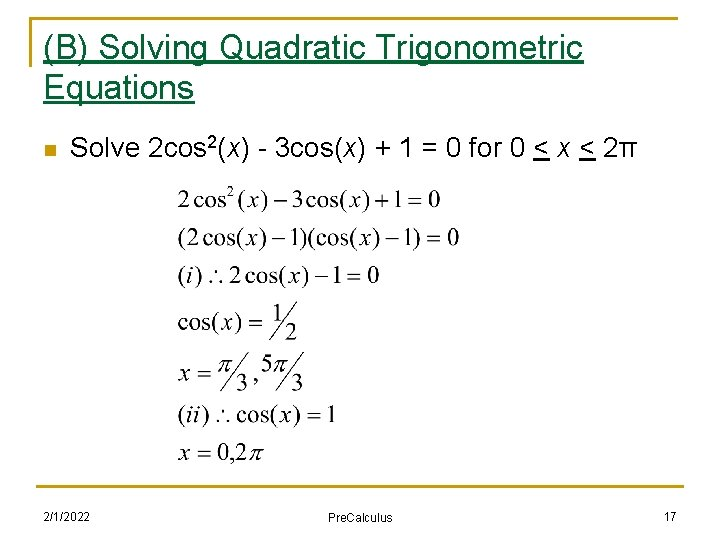(B) Solving Quadratic Trigonometric Equations n Solve 2 cos 2(x) - 3 cos(x) + 1 = 0 for 0 < x < 2π 2/1/2022 Pre. Calculus 17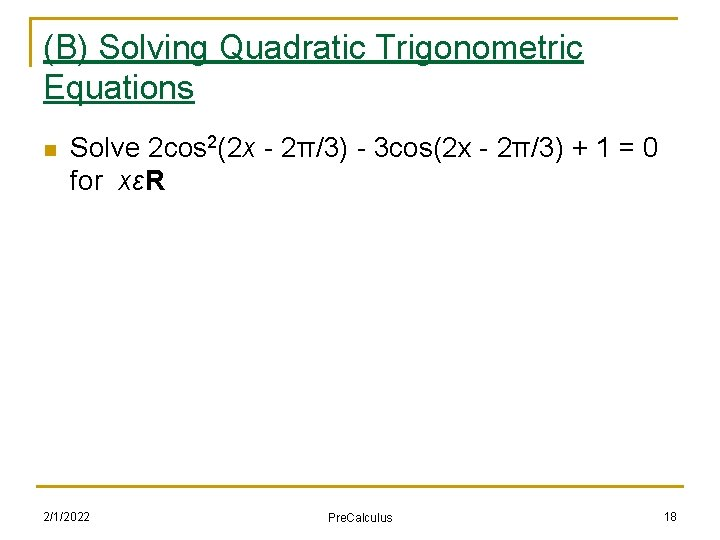(B) Solving Quadratic Trigonometric Equations n Solve 2 cos 2(2 x - 2π/3) - 3 cos(2 x - 2π/3) + 1 = 0 for xεR 2/1/2022 Pre. Calculus 18(C) Further Examples n Solve the following without a calculator 2/1/2022 Pre. Calculus 19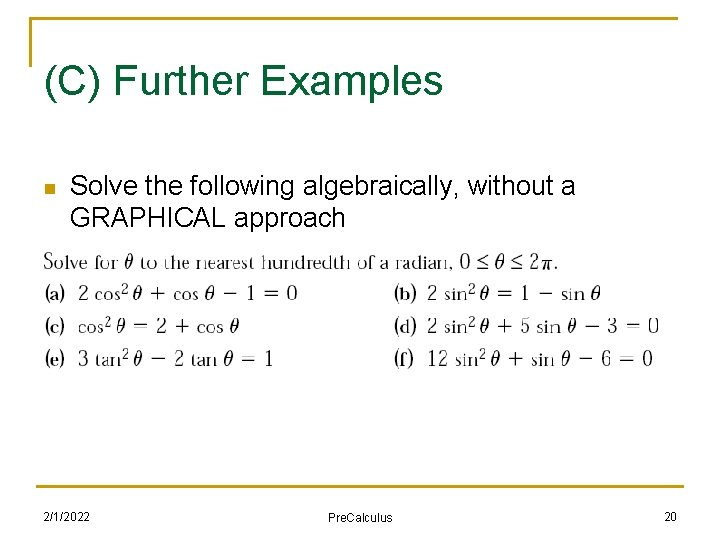(C) Further Examples n Solve the following algebraically, without a GRAPHICAL approach 2/1/2022 Pre. Calculus 20(D) Homework n Nelson Textbook, Chap 6. 6 n http: //mrsantowski. tripod. com/2010 Math. SLY 1/Ass essments/Nelson. S 66 p 541. pdf 2/1/2022 Pre. Calculus 21